# How to denote angle(∠) symbol in LaTeX?

In latex, the angel command is used by default to represent angel symbols. And angel command is the best practice for this symbol.

\documentclass{article}
\begin{document}
$\angle$
$\angle{ABC}$
\end{document}

Output :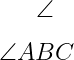And this angel command is also present in the asmsymb package.

\documentclass{article}
\usepackage{amssymb}
\begin{document}
$\angle{PQR}$
\end{document}

Output :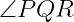This symbol is represented in two more ways according to the shape of the angle.

## Measure angel in LaTeX

Measure angle symbol is used to represent the size of angle. And this symbol is represented by the \measuredangle command that is present in both the amssymb and mathabx packages.

\documentclass{article}
\usepackage{amssymb}
\begin{document}
$\measuredangle{PQR}=30^{\circ}$
\end{document}

Output :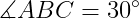And if you use the same command with mathabx package, there will be a slight difference in the shape of the symbol.

\documentclass{article}
\usepackage{mathabx}
\begin{document}
$\measuredangle{PQR}=45^{\circ}$
\end{document}

Output :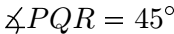## Spherical angel in LaTeX

There are no specific commands or packages to represent spherical angels. You can use more than one package and command. Notice this table below

 amssymb \sphericalangle →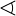wasysym \varangle →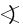mathabx \sphericalangle →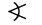marvosym \anglesign →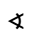\documentclass{article}
\usepackage{amssymb,wasysym}
\begin{document}
$\sphericalangle ABC$
$\varangle PQR$
\end{document}

Output :#### Md Jidan Mondal

LaTeX expert with over 10 years of experience in document preparation and typesetting. Specializes in creating professional documents, reports, and presentations using LaTeX.